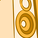# New to Qlik Sense

If you’re new to Qlik Sense, start with this Discussion Board and get up-to-speed quickly.

Announcements
Support Case Portal will move to Qlik Community Oct 4. Temporary case outage Oct 3. READ DETAILS
cancel
Showing results for
Did you mean:Contributor II

## Filter in Pivottable

Hi All,

I want  to put filter in Pivot table. I have the data where as i required where the value is more than >1000 and >-1000 that particular row should be displayed in table rest row not required. i used below expression but not able to filter the data

=sum({\$<[Variance classificaion]={'UP','UAP'>} [Far value difference])

+sum({\$<[Variance classificaion]={'UP','UAP'>} [booked price]).

Need help.

Its urgent

Binit Singh

Labels (1)
• ### Unknown

5 RepliesPartner
Hi Binitsingh,
Could you please give some small samples to show which kind of data is what you want or you don't want?
Thanks
AiolosContributor II
Author

Hi Aiolos,

Actually i dont have that access to share the data , but i am explaining you as per below scenario

Assume that we have two dimmension  and Three expression.

In expression we having three columns A,B,and C they contains some value. here we have to apply filter

1. if column A=1100 or -1100  or and B=0 nd C=0 then that  row should be displayed .

2. if column A=0  or and B=1100  or -1100 and C=0 then first row should be displayed.

if value is less than 1000 and -1000 that value should not be reflect.

Thnaks for reponse.

Binit.Partner
I think you can create some fake data to clarify these situations, so I can know which rows is what you want or not.Employee
It seems as if this question is not in the appropriate forum. This isn't a Data Catalyst question. Is it a Qlik Sense question?Contributor II
Author

Hi Ailos,

I have prepared one sample data for you on excel and prepared one QVW. There i created pivot Table my requirement is

Sum of daily income is '>=1000' or '>=-1000' that rows should be reflect.

I applied below formula:-

Below formula is working fine for positive value and value is displaying more than 1000 but for  negative its not coming

need your help to achieve >=-1000 value

=if(sum([Daily Income])>='1000',sum([Daily Income]))

Thanks

Binit SinghTags
Community Browser